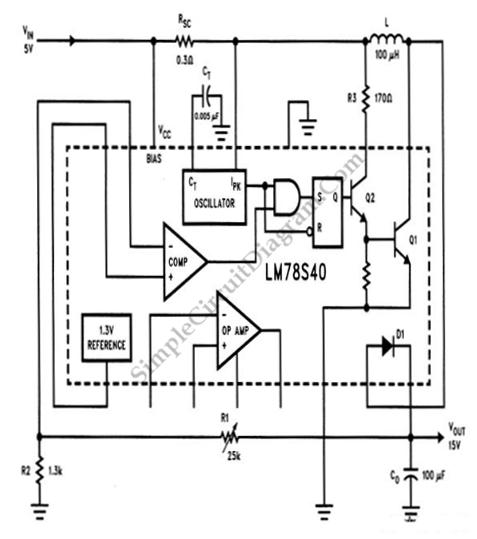# LM78S40 5V to 15V Step-up Voltage Regulator

We can do a step-up conversion without using transformer. Using this circuit, a 5V DC source can be converted into 15V. Please note that there is no power gain here, as we cannot create energy from nothing, it means that we can leverage the voltage but the current will drop. This circuit can provide 15V source but the maximum current will be limited to 150 mA (which is much lower than the supplied current at 5V input). The output has ripple less than 1% which is good enough for many applications. Here is the schematic diagram of the circuit: